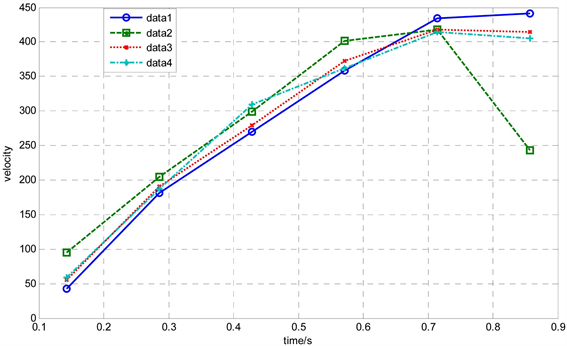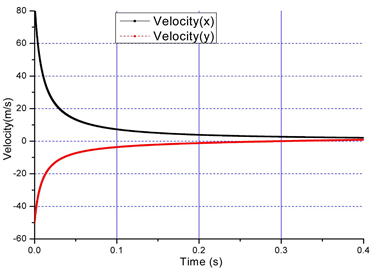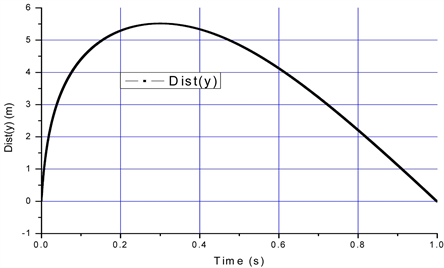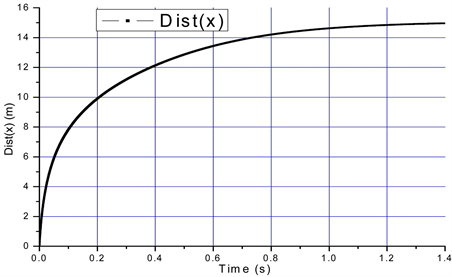﻿ 球类物体在空气中飞行阻力的应用研究 The Investigation about the Flying Drag Force of Sphere Type Body

Applied Physics
Vol. 09  No. 02 ( 2019 ), Article ID: 28800 , 7 pages
10.12677/APP.2019.92008

The Investigation about the Flying Drag Force of Sphere Type Body

Ning Xu*, Shanshui Wang, Ping Liu#

Civil Engineering and Architecture School, Jiangsu University of Science and Technology, Zhenjiang JiangsuReceived: Jan. 12th, 2019; accepted: Feb. 5th, 2019; published: Feb. 12th, 2019ABSTRACT

Due to the mechanical analysis of a flying bbadminton, some phenomena of the badminton sports were discussed and the theoretical explanation is presented. The experiments on the drag coefficient of the flying badminton were conducted. Based on the experiments, the badminton flight phenomenon was analyzed via the commercial software of Matlab. The results can be expanded into the other field and it can be added in the teaching process of fluid dynamic or the other class.

Keywords:Badminton, Drag Coefficient, Matlab, Numerical Analysis1. 引言

2. 控制方程Figure 1. The analytical layout of the flying badminton

$\left\{\begin{array}{l}m\frac{{\text{d}}^{2}{s}_{x}}{\text{d}{t}^{2}}=f\cdot \mathrm{cos}\theta \\ m\frac{{\text{d}}^{2}{s}_{y}}{\text{d}{t}^{2}}=f\cdot \mathrm{sin}\theta +mg\end{array}$ (1)

$\left\{\begin{array}{l}m\frac{\text{d}{v}_{x}}{\text{d}t}=-\frac{1.225}{2}{C}_{d}\left({v}_{x}^{2}+{v}_{y}^{2}\right)\frac{{v}_{x}}{\sqrt{\left({v}_{x}^{2}+{v}_{y}^{2}\right)}}\\ m\frac{\text{d}{v}_{y}}{\text{d}t}=-\frac{1.225}{2}{C}_{d}\left({v}_{x}^{2}+{v}_{y}^{2}\right)\frac{{v}_{y}}{\sqrt{\left({v}_{x}^{2}+{v}_{y}^{2}\right)}}+mg\end{array}$ (2)

$\frac{1.225{C}_{d}}{2m}=c$ ，则方程可化为：

$\left\{\begin{array}{l}\frac{\text{d}{v}_{x}}{\text{d}t}=-c{v}_{x}\sqrt{\left({v}_{x}^{2}+{v}_{y}^{2}\right)}\\ \frac{\text{d}{v}_{y}}{\text{d}t}=-c{v}_{y}\sqrt{\left({v}_{x}^{2}+{v}_{y}^{2}\right)}+g\end{array}$ (3)

$t+\text{const}=\frac{1}{2\sqrt{gc}}\mathrm{ln}|\frac{\sqrt{g/c}+{v}_{y}}{\sqrt{g/c}-{v}_{y}}|$

$t=\frac{1}{2\sqrt{gc}}\mathrm{ln}|\frac{\sqrt{g/c}+{v}_{y}}{\sqrt{g/c}-{v}_{y}}|$ (4)

${e}^{2\sqrt{gc}\cdot t}=\frac{\sqrt{g/c}+{v}_{y}}{\sqrt{g/c}-{v}_{y}}$

${s}_{y}={\int }_{0}^{T}2\sqrt{g/c}\frac{{e}^{2\sqrt{gc}\cdot t}}{1+{e}^{2\sqrt{gc}\cdot t}}-\sqrt{g/c}\text{\hspace{0.17em}}\text{d}t=\sqrt{g/c}\cdot t-{c}^{-1}\mathrm{ln}\left(\frac{1+{e}^{2\sqrt{gc}\cdot t}}{{e}^{2\sqrt{gc}\cdot t}}\right)$ (5)

3. 实验结果

4. 数值计算Figure 2. The plot of velocity vs timeFigure 3. The plot of height vs displacement (left) and velocity vs time (right)Figure 4. The plot of height vs timeFigure 5. The plot of displacement vs time

5. 结论

The Investigation about the Flying Drag Force of Sphere Type Body[J]. 应用物理, 2019, 09(02): 71-77. https://doi.org/10.12677/APP.2019.92008

1. 1. Li, Q., Guo, X., Qing, Q., et al. (2015) Dynamic Deflation Assessment of an Air Inflated Membrane Structure. Thin-Walled Structures, 94, 446-456. https://doi.org/10.1016/j.tws.2015.05.008

2. 2. Suo, H., Angelotti, A. and Zanelli, A. (2015) Thermal-Physical Behavior and Energy Performance of Air-Supported Membranes for Sports Halls: A Comparison among Traditional and Advanced Building Envelopes. Energy and Buildings, 109, 35-46. https://doi.org/10.1016/j.enbuild.2015.10.011

3. 3. Choi, Y. (2013) Analysis of Air-Inflated, Heavy, Membrane Tube Supported at Two Points on an Incline. European Journal of Mechanics-A/Solids, 42, 203-209. https://doi.org/10.1016/j.euromechsol.2013.05.005

4. 4. Bonet, J., Wood, R.D., Mahaney, J., et al. (2000) Finite Element Analysis of Air Supported Membrane Structures. Computer Methods in Applied Mechanics and Engineering, 190, 579-595. https://doi.org/10.1016/S0045-7825(99)00428-4

5. 5. 楚天都市报. 羽毛球速度世界最快? 傅海峰保持时速332公里纪录[EB/OL]. http://sports.sina.com.cn/o/2009-05-13/06004376733.shtml

7. 7. 郑植友, 周威. 中文体育类核心期刊羽毛球运动统计分析[J]. 成都体育学院学报, 2010, 36(2): 66-68.

8. 8. Wang, H., Zhai, Q. and Chen, K. (2019) Vortex-Induced Vibrations of an Elliptic Cylinder with Both Transverse and Rotational Degrees of Freedom. Journal of Fluids and Structures, 84, 36-55. https://doi.org/10.1016/j.jfluidstructs.2018.10.004

9. 9. Gu, Y., Du, J., Yang, D., et al. (2018) Form-Finding De-sign of Electrostatically Controlled Deployable Membrane Antenna Based on an Extended Force Density Method. Acta Astronautica, 152, 757-767. https://doi.org/10.1016/j.actaastro.2018.09.033

10. 10. De Nayer, G., Apostolatos, A., Wood, J.N., et al. (2018) Numerical Studies on the Instantaneous Fluid-Structure Interaction of an Air-Inflated Flexible Membrane in Turbulent Flow. Journal of Fluids and Structures, 82, 577-609. https://doi.org/10.1016/j.jfluidstructs.2018.08.005

11. 11. 周丽名. 动车组与普速列车交会空气动力学研究[D]. 成都: 西南交通大学, 2017.

12. 12. 柯世堂, 王同光, 曹九发, 等. 海上风力机随机风场模拟及风振响应分析[J]. 中南大学学报(自然科学版), 2016, 47(4): 1245-1252.

13. 13. Zhou, Y., Sun, Z. and Gao, Q. (2016) Performance-Based Fire Protection Design of Ruins Protection Pavilion Based on Air-Supported Membrane Structure. Procedia Engineering, 135, 486-494. https://doi.org/10.1016/j.proeng.2016.01.160

14. 14. Qing, Q. and Gong, J. (2015) Test Study and Numerical Simu-lation of Deflation Process of an Air-Supported Membrane Structure. Advances in Structural Engineering, 18, 761-774. https://doi.org/10.1260/1369-4332.18.6.761

15. 15. 吴望一. 流体力学(下) [M]. 北京: 北京大学出版社, 1983.

16. 16. 羽毛球之家. 如何选择羽毛球[EB/OL]. http://www.yumaoclub.com/special/shuttlecock_xuanze.htm

17. 17. 傅志忠. 羽毛球飞行特性初探[J]. 福建体育科技, 1990(2): 89-94.

18. NOTES

*第一作者。

#通讯作者。